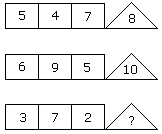# Verbal Reasoning - Character Puzzles - Discussion

### Discussion :: Character Puzzles - Character Puzzles 1 (Q.No.2)

2.

Which one will replace the question mark ?[A]. 1 [B]. 4 [C]. 3 [D]. 6

Explanation:

(5 + 4 + 7)/2 = 8

(6 + 9 + 5)/2 = 10

(3 + 7 + 2)/2 = 6.

 Priyanka said: (May 29, 2012) 1)5+7=12 12-4=8 2)6+9=15 15-5=10 3)7+2=9 9-3=6

 Santu Mondal said: (Jan 5, 2017) Thanks @Priyanka.

 Hira said: (Jun 2, 2017) 1) 5 4 7 Here 7 is the greatest number keep aside, add the rest (5+4)= 9 , the number in between 7 and 9 is 8. 2) 6 9 5 Similarly - 9 greatest keep aside add the rest (6+5) = 11, number in between 9 and 11 is 10, 3) Apply the same thing - 3 7 2 add the lowest number 3+2 = 5. Number between 5 and 7 is 6.

 Ruhulaminchoudhury said: (Jul 29, 2017) Smallest number among square * 2 givesthe answer is also possible. i.e 5 4 7, 4 *2 = 8 . 6 9 5then 5 * 2 = 10 therefore 3 7 2...2 * 2 = 4.

 Balu said: (Aug 5, 2017) Your explanation is awesome, Thanks @Hiri.

 Thanvi said: (Aug 7, 2017) Why it is divided by 2?

 Sulci said: (Nov 12, 2017) We can do 7-4 =3+5=8, in same way trick get apply then how can a question has 2 trick.

 Nishita Kadian said: (Aug 21, 2020) Good explanation, thanks @Hira.

 Monica said: (Dec 22, 2020) Thanks for the explanation @Hira.

 Shreyas Singh said: (Feb 10, 2021) 5+4+7 = 16 , half of 16 is 8. 6+9+5 = 20, half of 20 is 10. 3+7+2 = 12, half of 12 is 6.

 Sharukh said: (Jun 9, 2022) Super answer. Thanks.